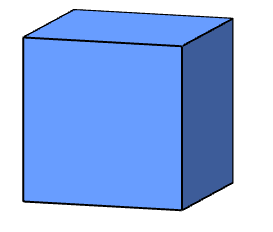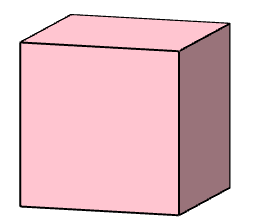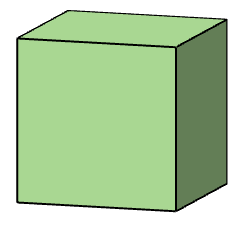#### You may also like### Some(?) of the Parts

A circle touches the lines OA, OB and AB where OA and OB are perpendicular. Show that the diameter of the circle is equal to the perimeter of the triangle### Ladder and Cube

A 1 metre cube has one face on the ground and one face against a wall. A 4 metre ladder leans against the wall and just touches the cube. How high is the top of the ladder above the ground?### At a Glance

The area of a regular pentagon looks about twice as a big as the pentangle star drawn within it. Is it?

# Three Cubes

##### Age 14 to 16Challenge Level1. The distance between centres of two adjacent faces of a cube is $8$cm.

What is the side length of the cube?2. I can make an equilateral triangle by cutting off the corner of a cube.

If the area of the largest equilateral triangle I can create in this way is $140$cm$^2$, what is the side length of this cube?3. A third cube has an edge length of $12$cm.

At each vertex, a tetrahedron with three mutually perpendicular edges of length $4$cm is cut away.

Can you find the volume and the surface area of the remaining solid?
•
•